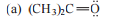Problem: What is the hybridization of oxygen or nitrogen in each of the following?

FREE Expert Solution
83% (426 ratings)
Problem Details

What is the hybridization of oxygen or nitrogen in each of the following?What scientific concept do you need to know in order to solve this problem?

Our tutors have indicated that to solve this problem you will need to apply the Hybridization concept. You can view video lessons to learn Hybridization Or if you need more Hybridization practice, you can also practice Hybridization practice problems .

What is the difficulty of this problem?

Our tutors rated the difficulty of What is the hybridization of oxygen or nitrogen in each of t... as low difficulty.

How long does this problem take to solve?

Our expert Organic tutor, Chris took 1 minute to solve this problem. You can follow their steps in the video explanation above.

What textbook is this problem found in?

Our data indicates that this problem or a close variation was asked in . You can also practice practice problems .